The known results on the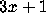problem are most elegantly expressed in terms of iterations of the function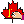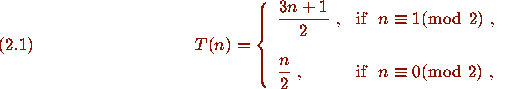One way to think of theproblem involves a directed graph whose vertices are the positive integers and that has directed edges from n to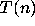. I call this graph the Collatz graph ofin honor of L. Collatz . A portion of the Collatz graph ofis pictured in Fig. 1. A directed graph is said to be weakly connected if it is connected when viewed as an undirected graph, i.e., for any two vertices there is a path of edges joining them, ignoring the directions on the edges. TheConjecture can be formulated in terms of the Collatz graph as follows.Annotation Form Interface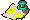```